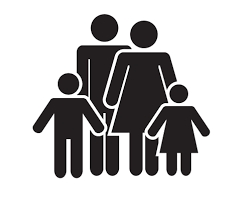# The father

The father is three times older than his son. 10 years ago, a father was seven times older than his son. How old are father and son?

o =  45
s =  15

### Step-by-step explanation:

o=3s
o-10 = 7(s-10)

o=3·s
o-10 = 7·(s-10)

o-3s = 0
o-7s = -60

Row 2 - Row 1 → Row 2
o-3s = 0
-4s = -60

s = -60/-4 = 15
o = 0+3s = 0+3 · 15 = 45

o = 45
s = 15

Our linear equations calculator calculates it.Did you find an error or inaccuracy? Feel free to write us. Thank you!

Tips for related online calculators
Do you have a system of equations and looking for calculator system of linear equations?
Do you want to convert time units like minutes to seconds?